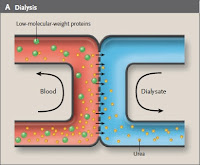# Clearance and Kt/VThe complete removal of a substance expressed as volume per unit time is known as clearance. The hemodialysis machine is built to be a urea clearance machine and typical urea clearance rates range from 200-260 ml/min, far more efficient than the native kidney urea clearance of around 65 ml/min.
In the nephrology world clearance of urea by the dialysis machine is expressed as K, which has units of ml/min. K is impacted by three factors:
1) The efficiency of the artificial kidney
2) The blood flow rate
3) The dialysate flow rate
Increasing each of the above increases K. The relationships are nonlinear. I had heard of the mysterious Kt/V prior to starting nephrology fellowship but knew nothing about it. It is a measure of dialysis adequacy that has a relationship to clinical outcome. K is, as above, the amount of urea completely cleared from blood in ml/min. t is time in minutes. V is the volume of distribution of urea in milliliters. So Kt/V is dimensionless.
Kt = (ml/min*min) = ml
V = ml
Kt/V = dimensionless ratio
NKF guidelines suggest a minimum Kt/V of 1.2 per hemodialysis session when three sessions per week are used in patients with no residual renal function based on cross sectional studies were increasing mortality has been associated with Kt/Vs of <1.2 and data from the HEMO trial.
In the HEMO trial patients were randomized in a 2×2 factorial design to high or low flux dialysis membranes and Kt/V targets of 1.25 or 1.65 for each session of three times per week hemodialysis. There was no difference in the primary endpoint of mortality between any of these main groups. (The Kt/Vs above are the single pool Kt/Vs – more on this in the future.)
For a quick idea of what a Kt/V of 1.2 means in terms of urea clearance (K) over the course of the week let’s run the numbers in a 75kg male dialyzing three times a week for three hours each session…
Kt/V = 1.2
V = volume of distribution of urea in a male = 0.55*75kg = 41.25L = 41,250ml
K = 1.2(V)/t = 1.2(41,250ml) = 49,500ml/t
For the week 3*49,500ml/t = 148,500ml/t
t = 10,080min/week
148,500ml/10,080min = 14.7ml/min
So although the hemodialysis machine is incredibly efficient during the time it is operating it’s clearance averaged over the course of a week in traditional three times a week dialysis is much less impressive.

1.Thank you for the very good explanation.
I was wondering if you may have a hint on how to estimate the Kt/V of native kidneys for a day.
Thanks a lot, best regards, Michaela

2.BUN is the mass of nitrogen within urea/volume, not the mass of urea….

3.How can you get the value of K in Kt/V?

4.How do you calculate kt/v

5.Do you ever calculate the patient's residual urea clearance with the KT/V in patients whose KT/V is suboptimal? We used to do that years ago, but I don't see it done today. I also can't remember the calculation.
Thanks

6.The Urea Reduction Ratio (URR) is calculated as follows:

(pre dialysis BUN – post dialysis BUN)/pre dialysis BUN

The ratio is often multiplied by 100 to give a percent.

7.how do u calculate URR?

8.Good question (I always have to work it out from scratch):

1 hr = 60 min
24 hrs = 1 day
7 days = 1 week
24hrs*60min= 1440min in a day
1440*7= 10,080min in a week

9.How did you get that many minutes per week?